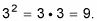##### Basic Math & Pre-Algebra All-in-One For Dummies (+ Chapter Quizzes Online)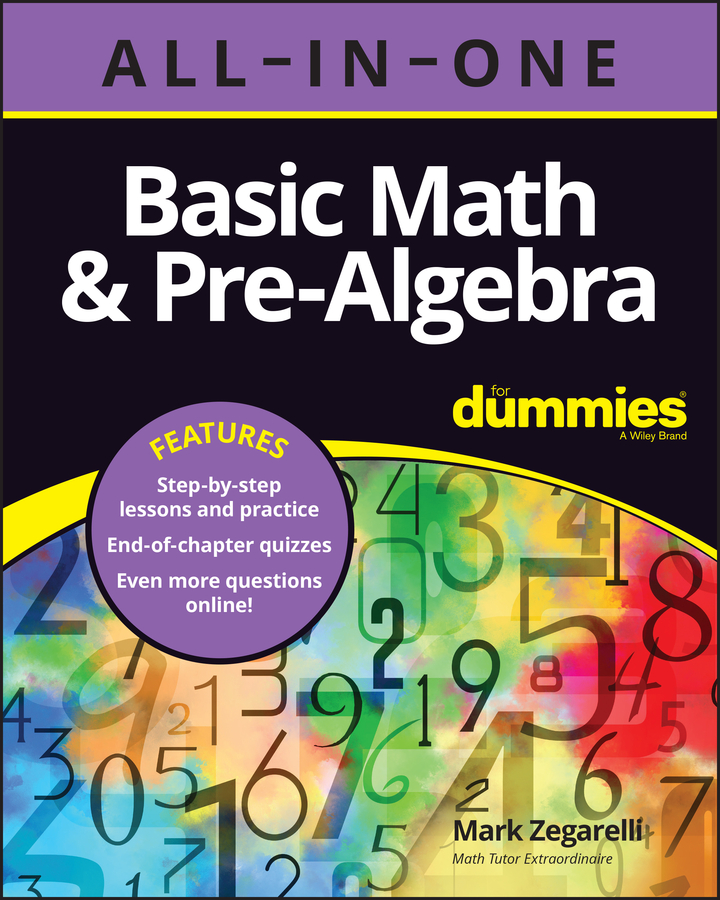Radicals and exponents (also known as roots and powers) are two common — and oftentimes frustrating — elements of basic algebra. And of course they follow you wherever you go in math, just like a cloud of mosquitoes follows a novice camper. The best thing you can do to prepare for calculus is to be ultra-solid on what can and can’t be done when simplifying with exponents and radicals. You’ll want to have this knowledge so that when more challenging math problems come along, the correct answers come along also.

Here are some important facts about what radicals and exponents are and how they relate to each other:

• A radical is a root of a number. Radicals are represented by the root sign,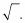For example, if you take the 2nd root of the number 9 (or the square root), you get 3 because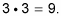If you take the 3rd root (or the cube root) of 64, you get 4 because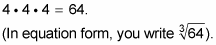The square root of any number represents the principal root (the fancy term for the positive root) of that number. For example,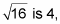even though (–4)2 gives you 16 as well.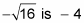because it’s the opposite of the principal root. When you’re presented with the equation x2 = 16, you have to state both solutions: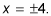Also, you can’t take the square root of a negative number; however, you can take the cube root of a negative number. For example, the cube root of –8 is –2, because (–2)3 = –8.

• An exponent represents the power of a number. If the exponent is a whole number — say, 2 — it means the base is multiplied by itself that many times — two times, in this case. For example,